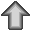# Calculation of the representation factor and the associated probability

Two groups of genes are compared and found to have x genes in common. A representation factor and the probability of of finding an overlap of x genes are calculated.

### Representation factor

The representation factor is the number of overlaping genes divided by the expected number of overlaping genes drawn from two independent groups.

A representation factor > 1 indicates more overlap than expected of two independent groups, a representation factor < 1 indicates less overlap than expected, and a representation factor of 1 indicates that the two groups by the number of genes expected for independent groups of genes.

x = # of genes in common between two groups.
n = # of genes in group 1.
D = # of genes in group 2.
N = total genes, in this case the 17611 genes with good spots on the Kim lab full genome chips.
C(a,b) is the number of combinations of a things taken b at a time.

The representation factor = x / expected # of genes.
Expected # of genes = (n * D) / N

### Probability

#### Exact hypergeometric probability

The probability of finding x overlapping genes can be calculated using the hypergeometric probability formula:

C(D, x) * C(N-D, n-x) / C(N,n)

If x is less than the expected number of overlapping genes, the probability of finding x or fewer genes is:

Prob = sum (i=0 to i=x) [ C(D, i) * C(N-D, n-i) / C(N,n) ]

If x is greater than the expected number of overlapping genes, the probability of finding x or more genes is:

Prob = 1- sum (i=0 to i=(x-1)) [ C(D, i) * C(N-D, n-i) / C(N,n) ]

C(a,b) is calculated using:

C(a,b) = a! / ((a - b)! * b!)
C(a,b) = exp(gammln(a + 1)-gammln(1+(a - b))-gammln(b + 1))

gamma(a + 1) = a!.
and gammln(a + 1) is an approximation of a! calculated using code from Chapter 6.1 of Numerical Recipes in C: The Art of Scientific Computing (ISBN 0-521-43108-5)

#### Normal approximation

The exact hypergeometric probability is difficult to calculate, so a normal approximation is used when:

p +/- 2*sqrt(p*q/n) is > 0 and n * 10 < N.

where p = D / N and q = 1 - p.

The normal approximation is:

Z = abs (((x-.5) - n * p) / sqrt(n * p * q)).

Prob = P { Z } where Z is a standard normal variate from N(0,1). P { Z } = 1 - ((erff(Z / sqrt(2)) + 1) / 2
where erff is the error function calcuated using code from Chapter 6.2 of Numerical Recipes in C: The Art of Scientific Computing (ISBN 0-521-43108-5)Return to the home page

Please send comments or questions regarding this web page to Jim Lund (jlund256 at gmail dot com)